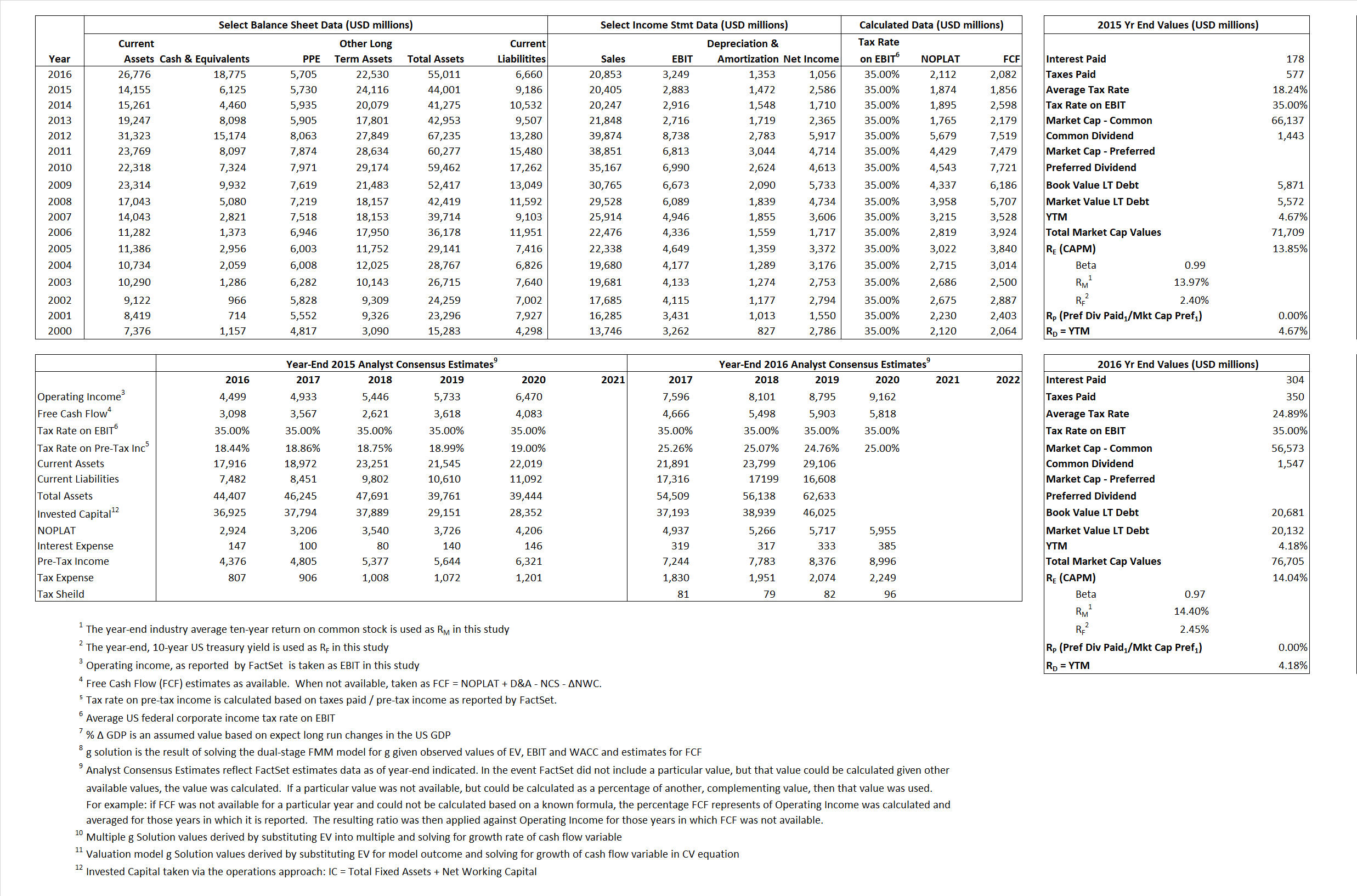# Abbot Laboratories

## Analyst Listing

The following analysts provide coverage for the subject firm as of May 2016:

 Broker Analyst Analyst Email BTIG Dane Leone dleone@btig.com Leerink Partners Danielle Antalffy danielle.antalffy@leerink.com Argus Research David Toung dtoung@argusresearch.com Morningstar Debbie S. Wang debbie.wang@morningstar.com RBC Capital Markets Glenn Novarro glenn.novarro@rbccm.com Erste Group Hans Engel hans.engel@erstegroup.com Raymond James Jayson Bedford jayson.bedford@raymondjames.com Jefferies Jeffrey Holford jholford@jefferies.com Gabelli & Company Jennie Tsai jtsai@gabelli.com BMO Capital Markets Joanne K. Wuensch joanne.wuensch@bmo.com Cowen & Company Joshua Jennings josh.jennings@cowen.com First Global Stockbroking Kavita Thomas kavita.thomas@fglobal.com Deutsche Bank Research Kristen Stewart kristen.stewart@db.com Wells Fargo Securities Larry Biegelsen lawrence.biegelsen@wellsfargo.com William Blair Margaret Kaczor mkaczor@williamblair.com Credit Suisse Matthew Keeler matthew.keeler@credit-suisse.com Stifel Nicolaus Rick Wise wiser@stifel.com

## Primary Input Data## Derived Input Data

### Equational Form

Net Operating Profit Less Adjusted Taxes NOPLAT  1,874 2,112$NOPLAT\, =\, EBIT\, x\, (1 \,-\, Avg \,\,Tax\,\, Rate\,\, on\,\, EBIT)$
Free Cash Flow FCF 1,856 2,082$FCF\,=NOPLAT\,+\,Non-Cash\,Expenses-\Delta NWC\,-\,NCS$
Tax Shield TS 32 76$TS\,=\,Interest\,\,Paid\,\,x\,\, Avg \,\,Tax\,\,Rate\,\, on\,\, Pre-Tax\,\, Income$
Invested Capital IC 34,815 48,351$IC\,=\,Fixed\,\,Operating\,\,Assets\,\,+\,\,Net\,\, Working\,\, Capital$
Return on Invested Capital ROIC 5.38% 4.37%$ROIC\,=\,\frac { NOPLAT }{ IC }$
Net Investment NetInv 5,544 14,889$NetInv\,=\,{ {IC}_{1}}-{{IC}_{0}}+Depreciation$
Investment Rate IR 295.85% 705.02%$IR\,=\,\frac {NetInv}{NOPLAT}$
Weighted Average Cost of Capital
WACCMarket  13.07%  11.18%$WACC\,=\,\frac { E }{ V } { R }_{ E }\,+\,\frac { P }{ V } { R }_{ P }\,+\,\frac { D }{ V } { R }_{ D }\left( 1- Avg\,\, Tax\,\,Rate\,\,on\,\,Pre-Tax\,\,Income \right)$
WACCBook  8.46%  6.96%
Enterprise value
EVMarket  65,584  57,930$EV\,=\,Market\,\,Cap\,\,Equity\,+\,\,Long\,\,Term\,\,Debt\,-\,Cash$
EVBook  65,883  58,479
Long-Run Growth
g = IR x ROIC
15.92%  30.79% Long-run growth rates of the income variable are used in the Continuing Value portion of the valuation models.
g = %$\Delta$ GDP  2.50%  2.50%
Margin from Operations M  14.13%  15.58%$M\,\,=\,\,\frac{EBIT}{SALES}$
Depreciation/Amortization Rate D 33.80%  29.40%$D\,\,=\,\,\frac{D+A}{EBITDA}$

## Valuation Multiple Outcomes

The outcomes presented in this study are the result of original input data, derived data, and synthesized inputs.

### model g solution

12/31/2015 12/31/2016 12/31/2015 12/31/2016 12/31/2015 12/31/2016

EV/SALES$\frac {EV}{Sales} \,= \,\frac{ROIC\, -\, g}{ROIC\,(WACC\,-\,g)}\,(1\,-\,T)\,(M)$

3.21  2.78  21.78% 45.57%  17.22% 31.70%

EV/EBITDA$\frac {EV}{EBITDA} \,= \,\frac{ROIC\, -\, g}{ROIC\,(WACC\,-\,g)}\,(1\,-\,T)\,(1\,-\,D)$

15.06  12.59 21.78%  45.57% 17.22%  31.70%

EV/NOPLAT$\frac {EV}{NOPLAT} \,= \,\frac{ROIC\, -\, g}{ROIC\,(WACC\,-\,g)}$

35.00  27.43 21.78%  45.57% 17.22%  31.70%

EV/FCFOPS$\frac {EV}{FCF_{OPS}} \,= \,\frac{ROIC\, -\, g}{ROIC\,(WACC\,-\,g)}\,(1\,-\,T)$

35.34 27.82 21.78%  45.57% 17.22%  31.70%

EV/EBIT$\frac {EV}{EBIT} \,= \,\frac{ROIC\, -\, g}{ROIC\,(WACC\,-\,g)}\,(1\,-\,T)$

22.75  17.83 21.78%  45.57% 17.22%  31.70%

EV/IC$\frac {EV}{IC} \,= \,\frac{ROIC\, -\, g}{WACC\,-\,g}$

1.88   1.20 21.78%  45.57% 17.22%  31.70%